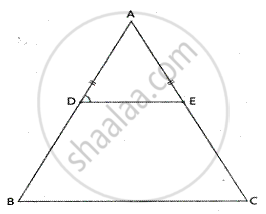Share

D and E Are Points on Equal Sides Ab and Ac of an Isosceles Triangle Abc Such that Ad = Ae. Prove that the Points B, C, E and D Are Concyclic. - ICSE Class 10 - Mathematics

Question

D and E are points on equal sides AB and AC of an isosceles triangle ABC such that AD = AE. Prove that the points B, C, E and D are concyclic.

SolutionGiven – In ∆ABC, AB = AC and D and E are points on AB and AC
Such that AD = AE. DE is joined.
To prove B, C, E, D are concyclic.

Proof – In ∆ABC, AB = AC

∴ ∠B = ∠C     [Angles opposite to equal sides]

Similarly, In ∆ADE, AD = AE      [Given]

∴ ∠ADE = ∠AED   [Angles opposite to equal sides]
In ∆ABC,

∴ (AD)/(AB) = (AE )/(AC)
DE || BC
∴ ∠ADE = ∠B      [corresponding angles]
But ∠B = ∠C      [proved]

∴ Ext ∠ADE = its interior opposite ∠C
∴ BCED is a cyclic quadrilateral
Hence B, C, E and D are concyclic.

Is there an error in this question or solution?
Solution D and E Are Points on Equal Sides Ab and Ac of an Isosceles Triangle Abc Such that Ad = Ae. Prove that the Points B, C, E and D Are Concyclic. Concept: Cyclic Properties.
S